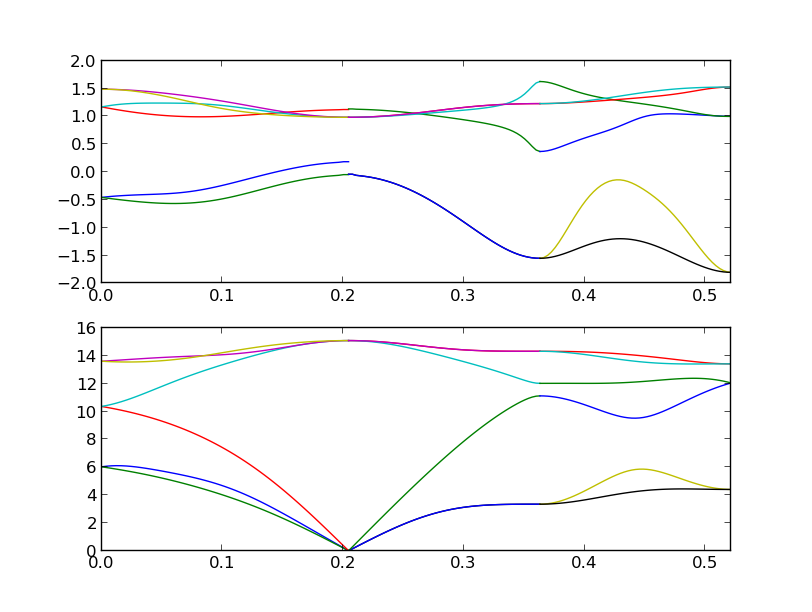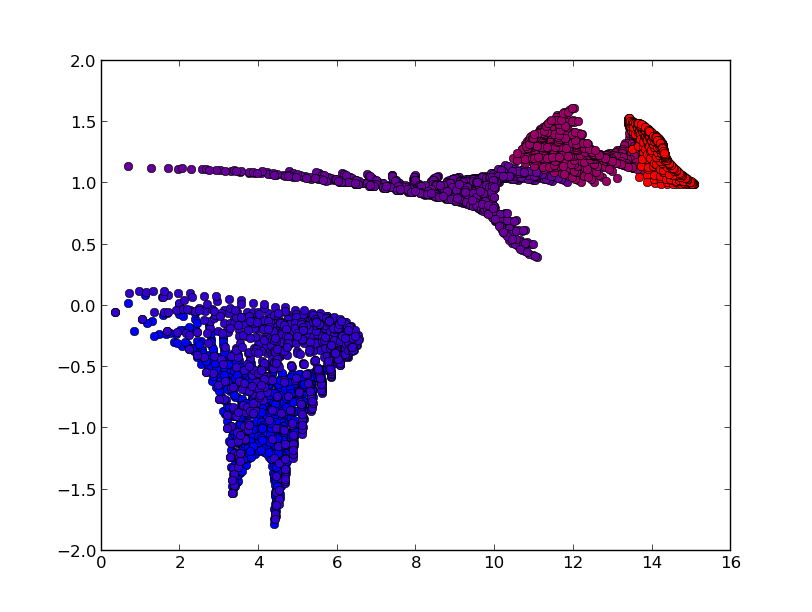# Calculation of mode Grüneisen parameters#

## How to run#

It is necessary to run three phonon calculations. One is calculated at the equilibrium volume and the remaining two are calculated at the slightly larger volume and smaller volume than the equilibrium volume. The unit cells at these volumes have to be fully relaxed under the constraint of each volume.

Let files named POSCAR-unitcell, FORCE_SETS (or FORCE_CONSTANTS with --readfc option), and optionally BORN stored in three different directories named, e.g., equiv, plus, and minus.

The calculated results are written into the file gruneisen.yaml.

In the example directory, an example of silicon (Si-gruneisen) is prepared. A calculation along paths in reciprocal space can be made by

% phonopy-gruneisen orig plus minus --dim="2 2 2" --pa="0 1/2 1/2 1/2 0 1/2 1/2 1/2 0" --band="1/2 1/4 3/4 0 0 0 1/2 1/2 1/2 1/2 0.0 1/2" -p -c POSCAR-unitcellIn this calculation, neighboring q-points in each band segment are connected considering their phonon symmetry to treat band crossing correctly. Therefore the phonon frequencies may not be ordered in gruneisen.yaml. In the plot (-p option), the colors of phonon bands correspond to those of mode Grüneinen parameters.

A calculation on a reciprocal mesh is made by

% phonopy-gruneisen orig plus minus --dim="2 2 2" --pa="0 1/2 1/2 1/2 0 1/2 1/2 1/2 0" --mesh="20 20 20" -p -c POSCAR-unitcell --color="RB"In the plot (-p option), the colors of mode Grüneinen parameters are set for band indices with ascending order of phonon frequencies.

Mode Grüneinen parameter may diverge around $$\Gamma$$-point. In the above example for band paths, mode Grüneinen parameters are calculated at $$\Gamma$$-point, but phonopy-gruneisen script avoids showing the values on the plot. Instead the values at the neighboring q-points of $$\Gamma$$-point are used for the plot.

## Force calculator interfaces#

Options of force calculator interfaces such as --abinit, --qe, … can be specified for corresponding calculators and the crystal structure file format should be different from that of the VASP format. An Abinit example is as follows:

% phonopy-gruneisen orig plus minus --abinit --dim="2 2 2" --pa="0 1/2 1/2 1/2 0 1/2 1/2 1/2 0" --band="1/2 1/4 3/4 0 0 0 1/2 1/2 1/2 1/2 0.0 1/2" -p -c Si.in


## Command options#

If one of the force calculator options is specified, the interface mode is changed to it. The unit conversion factor to THz is appropriately selected and its crystal structure file format is accepted. If none of them is specified, as the VASP interface mode is invoked as the default interface.

The following command options can be used for all interface modes. They work similarly to those for phonopy script.

• --dim

• --mp, --mesh

• --band

• --pa, --primitive_axis

• --readfc

• --band_points

• --nac

• --factor

• --nomeshsym

• -p

• -c

• -s, --save

• -o

Currently --pa="auto" works but --band="auto" doesn’t. The --color option (RB, RG, RGB) is used to gradually change the marker colors with respect to band indices. For the mesh-sampling plot, a few more options to control matplotlib parameters are prepared.

## Method to calculate mode Grüneisen parameters#

Mode Grüneisen parameter $$\gamma(\mathbf{q}\nu)$$ at the wave vector $$\mathbf{q}$$ and band index $$\nu$$ is given by

$\begin{split}\gamma(\mathbf{q}\nu) =& -\frac{V}{\omega(\mathbf{q}\nu)}\frac{\partial \omega(\mathbf{q}\nu)}{\partial V}\\ =&-\frac{V}{2[\omega(\mathbf{q}\nu)]^2}\left<\mathbf{e}(\mathbf{q}\nu)\biggl| \frac{\partial D(\mathbf{q})} {\partial V}\biggl|\mathbf{e}(\mathbf{q}\nu)\right>,\end{split}$

where $$V$$ is the volume, $$\omega(\mathbf{q}\nu)$$ is the phonon frequency, $$D(\mathbf{q})$$ is the dynamical matrix, and $$\mathbf{e}(\mathbf{q}\nu)$$ is the eigenvector. This is approximated by the finite difference method:

$\gamma(\mathbf{q}\nu) \simeq -\frac{V}{2[\omega(\mathbf{q}\nu)]^2} \left<\mathbf{e}(\mathbf{q}\nu)\biggl| \frac{\Delta D(\mathbf{q})} {\Delta V}\biggl|\mathbf{e}(\mathbf{q}\nu)\right>.$

The phonopy-gruneisen script requires three phonon calculations at corresponding three volume points. One is for eigenvectors at the equilibrium volume ($$V$$) and the remaining two are for $$\Delta D(\mathbf{q})$$ with slightly larger and smaller volumes than $$V$$.